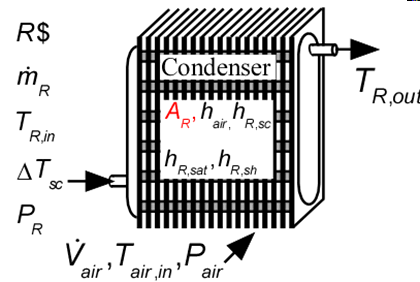Contents

Condenser1_CLCondenser1_CL determines the required heat transfer surface area to condense a superheated refrigerant to a specified degree of subcooling.  Thermal energy is rejected to an air stream.  It is necessary to provide the heat transfer coefficients on the air side of the heat exchanger and for the superheated, saturated, and subcooled sections on the refrigerant side.  These heat transfer coefficients can be determined using the functions in the Heat Transfer Library, as illustrated in the example. See Condenser3_CL for a similar routine in which the heat transfer coefficients are calculated internally. EvaporativeCondenser1_CL provides a similar routine for sizing an evaporative condenser.

This library file can be used in the design stage of the condenser by inputing the design inlet conditions to determine the required condenser size.  Use Condenser2_CL to determine the refrigerant and air outlet states for given inlet conditions and condenser area.

Inputs:

R\$:   name of the refrigerant

m_dot_R:  refrigerant flow rate (kg/s, lbm/hr)

h_R_in:  specific enthalpy of entering refrigerant (J/kg, kJ/kg, Btu/lbm)

DELTAT_sc:  number of degrees of subcooling (C, K, F, R)

P_R:  refrigerant pressure (Pa, kPa, bar, MPa, psia, atm)

V_dot:  volumetric flow rate of air (m^3/s, cfm)

T_air_in:  air inlet temperature  (C, K, F, R)

P_air:  air pressure (Pa, kPa, bar, MPa, psia, atm)

h_air:  effective outside (air) heat transfer coefficient per unit refrigerant tube area (W/m^2-K, Btu/hr-ft^2-R)

A_air\A_R:  ratio of effective heat transfer area on the air side to the heat transfer area for the refrigerant*

h_R_SH:  heat transfer coefficient for refrigerant in the superheat regime  (W/m^2-K, Btu/hr-ft^2-R)

h_R_sat:  average heat transfer coefficient for refrigerant in the saturated regime  (W/m^2-K, Btu/hr-ft^2-R)

h_SC:  average heat transfer coefficient for refrigerant in the saturated regime  (W/m^2-K, Btu/hr-ft^2-R)

Outputs:

A_R:  required heat transfer area for the refrigerant (m^2, ft^2)

UA:  overall heat transfer coefficient (W/K ,Btu/hr-R)

Q_dot:  overall heat transfer rate (W, kW, Btu/hr)

h_R_out refrigerant outlet specific enthalpy (J/kg, kJ/kg, Btu/lbm)

T_air_out:  outlet temperature of the air  (C, K, F, R)

f_sh:  fraction of the condenser area that is in the superheat regime

f_sc:  fraction of condenser area that is in the subcooled regime

*A_air\A_R is the ratio of the product of the overall fin efficiency and total (finned and unfinned) area on the air side to the total surface area inside the refrigerant flow passages.  For unfinned surfaces, A_air\A_R is the ratio of the outer surface to inner surface areas.

Example:

\$unitSystem SI C kPa kJ mass

\$TabStops 0.2 4.5 in

\$VarInfo A_tot Lower=0.1  Upper=10  units=m^2

\$VarInfo alpha units=1/m

\$VarInfo D_h units=m

\$VarInfo D_in units=m

\$VarInfo D_o units=m

\$VarInfo h_air1 units=W/m^2-K

\$VarInfo h_cond units=W/m^2-K

\$VarInfo h_R_in units=kJ/kg

\$VarInfo h_R_out units=kJ/kg

\$VarInfo Q_dot units=kW

\$VarInfo T_air_out units=C

\$VarInfo T_w units=C

\$VarInfo UA units=W/K

\$VarInfo A_finned units=m^2

\$VarInfo A_unfinned units=m^2

\$VarInfo A_fr units=m^2

\$VarInfo fin_thk units=m

\$VarInfo m_dot_air units=kg/s

\$VarInfo h_T_sc units=W/m^2-K

\$VarInfo T_R_sat units=C

\$VarInfo A_R units=m^2

\$VarInfo DELTAP_sc units=kPa

\$VarInfo DELTAP_sh units=kPa

\$VarInfo fin_pitch units=1/m

\$VarInfo h_h_sc units=W/m^2-K

\$VarInfo h_h_sh units=W/m^2-K

\$VarInfo h_T_sh units=W/m^2-K

R\$='R134a' "refrigerant"

P_R=1 [MPa]*convert(MPa,kPa) "refrigerant pressure"

m_dot_R=0.0028 [kg/s] "refrigerant mass flow rate"

T_R_in=95 [C]

h_R_in=enthalpy(R\$,T=T_R_in,P=P_R) "inlet refrigerant temperature"

DELTAT_sc=4 [C] "subcooling"

V_dot_air=0.06 [m^3/s] "volumetric flow rate of air"

T_air_in=20 [C] "air inlet temperature"

P_air=1 [atm]*convert(atm,kPa) "air pressure"

h_air=44.3 [W/m^2-K] "convection coefficient for air.  See h_air1 calculation below"

A_air\A_R=12.13 [-] "ratio of air on air side to refrigerant side.  See A_air\A_R1 calculation"

h_R_sh=170 [W/m^2-K] "convection coefficient for superheated R134a.  See h_H_sh calculation"

h_R_sat=1332 [W/m^2-K] "convection coefficient for saturated R134a.  See h_cond calculation"

h_R_SC=130 [W/m^2-K] "heat transfer coefficient in subcooled section.  See h_H_sc calculation"

Call condenser1_cl(R\$, m_dot_R, h_R_in, DELTAT_sc, P_R, V_dot_air, T_air_in, P_air, h_air, A_air\A_R, h_R_sh, h_R_sat, h_R_sc:  A_R, UA, Q_dot, h_R_out, T_air_out, f_sh, f_sc)

"!Calculation of the heat transfer coefficients using the Heat Transfer Library functions"

N_circuits=1 "number of parallel circuits"

A_fr=0.26*0.2 [m^2] "frontal area"

W=0.2 [m] "length of tubes"

N_tubes=A_R/(pi*W*D_in) "required number of tubes"

Call chx_geom_finned_tube('fc_tubes_s80-38T': D_o, fin_pitch, D_h, fin_thk, sigma, alpha, A_fin\A) "air side geometry"

"Determine ratio of area on air side to the refrigerant heat transfer area"

th = 0.9 [mm]*convert(mm,m) "tube wall thickness"

D_in=D_o-2*th "inner tube diameter"

A_unfinned=pi*D_o*N_tubes*W*(1-fin_thk*fin_pitch) "unfinned area on air side"

A_tot=A_unfinned+A_finned "total finned and unfinned area on air side"

A_finned/A_tot=A_fin\A "ratio of finned to total area is provided by Compact HX library"

eta_o=0.97 "overall fin efficiency - See Heat Transfer, Nellis and Klein, section 8.5"

A_air\A_R1=eta_o*A_tot/(N_tubes*W*pi*D_in) "ratio of heat transfer area on air side to that on refrigerant side"

"Determine heat transfer coefficients for air and refrigerant "

T_R_sat=t_sat(R\$,P=P_R)

m_dot_air=V_dot_air/volume(Air,T=T_air_in,P=P_air) "air mass flow rate"

Call chx_h_finned_tube('fc_tubes_s80-38T', m_dot_air, A_fr, 'Air', T_air_in, P_air: h_air1) "compact hx correlation"

Call pipeflow('R134a',T_R_in, P_R, m_dot_R/N_circuits, D_in, N_tubes*W*f_sh, 0: h_T_sh, h_H_sh, DELTAP_sh, Nusselt_T_sh, f_R_sh, Re_R_sh) "superheat section"

T_w=T_air_in "estimated wall temperature"

Call cond_horizontaltube_avg(R\$, m_dot_R/N_circuits, T_R_sat, T_w, D_in, 1, 0 : h_cond) "saturated section"

Call pipeflow('R134a',T_R_sat-1 [C], P_R, m_dot_R/N_circuits, D_in, N_tubes*W*f_sc, 0: h_T_sc, h_H_sc, DELTAP_sc, Nusselt_T_sc, f_R_sc, Re_R_sc) "subcooled section"

{Solution:

A_R=0.1303 [m^2]

f_sc=0.07965

f_sh=0.2645

h_R_out=101.4 [kJ/kg]

Q_dot=0.6397 [kW]

T_air_out=28.81 [C]

UA=38.25 [W/K]

}